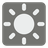Sun Angle
Simple
English JA RU

Every true traveler must know how to do 3 things: fix the fire, find the water and extract useful information from the nature around him. Programming won't help you with the fire and water, but when it comes to the information extraction - it might be just the thing you need.

Your task is to find the angle of the sun above the horizon knowing the time of the day. Input data: the sun rises in the East at 6:00 AM, which corresponds to the angle of 0 degrees. At 12:00 PM the sun reaches its zenith, which means that the angle equals 90 degrees. 6:00 PM is the time of the sunset so the angle is 180 degrees. If the input will be the time of the night (before 6:00 AM or after 6:00 PM), your function should return - "I don't see the sun!".Input: The time of the day.

Output: The angle of the sun, rounded to 2 decimal places.

Example:

```sun_angle("07:00") == 15
sun_angle("12:15"] == 93.75
sun_angle("01:23") == "I don't see the sun!"```

How it is used: One day it can save your life, if you'll be lost far away from civilization.

Precondition:
00:00 <= time <= 23:59